# RS Aggarwal Solutions for Class 8 Chapter 2 - Exponents Exercise 2c

The pdf containing the RS Aggarwal Solutions of the Exercise 2C is available here. Our experts have solved the RS Aggarwal Solutions to ensure that the students are thorough with their concepts by practising the solutions. In this exercise, students study the concepts related to laws of exponents and the method of converting given numbers into standard form. They also learn about the positive and negative integral exponent of a rational number. RS Aggarwal textbook has plenty of questions in it, we advise the students to go through the link given below. By practising the RS Aggarwal Solutions for class 8, students will be able to grasp the concepts perfectly.

## Download PDF of RS Aggarwal Solutions for Class 8 Chapter 2 – Exponents Exercise 2C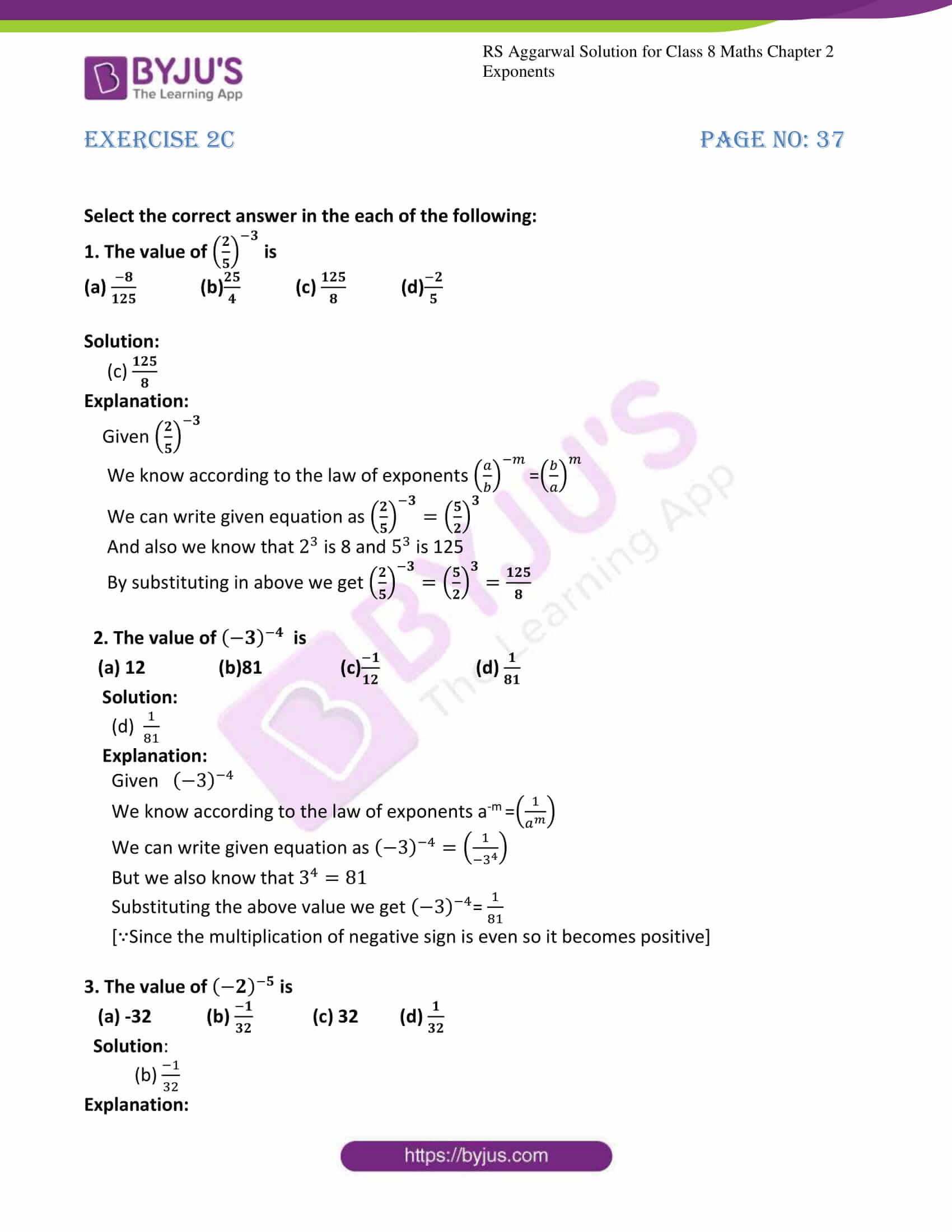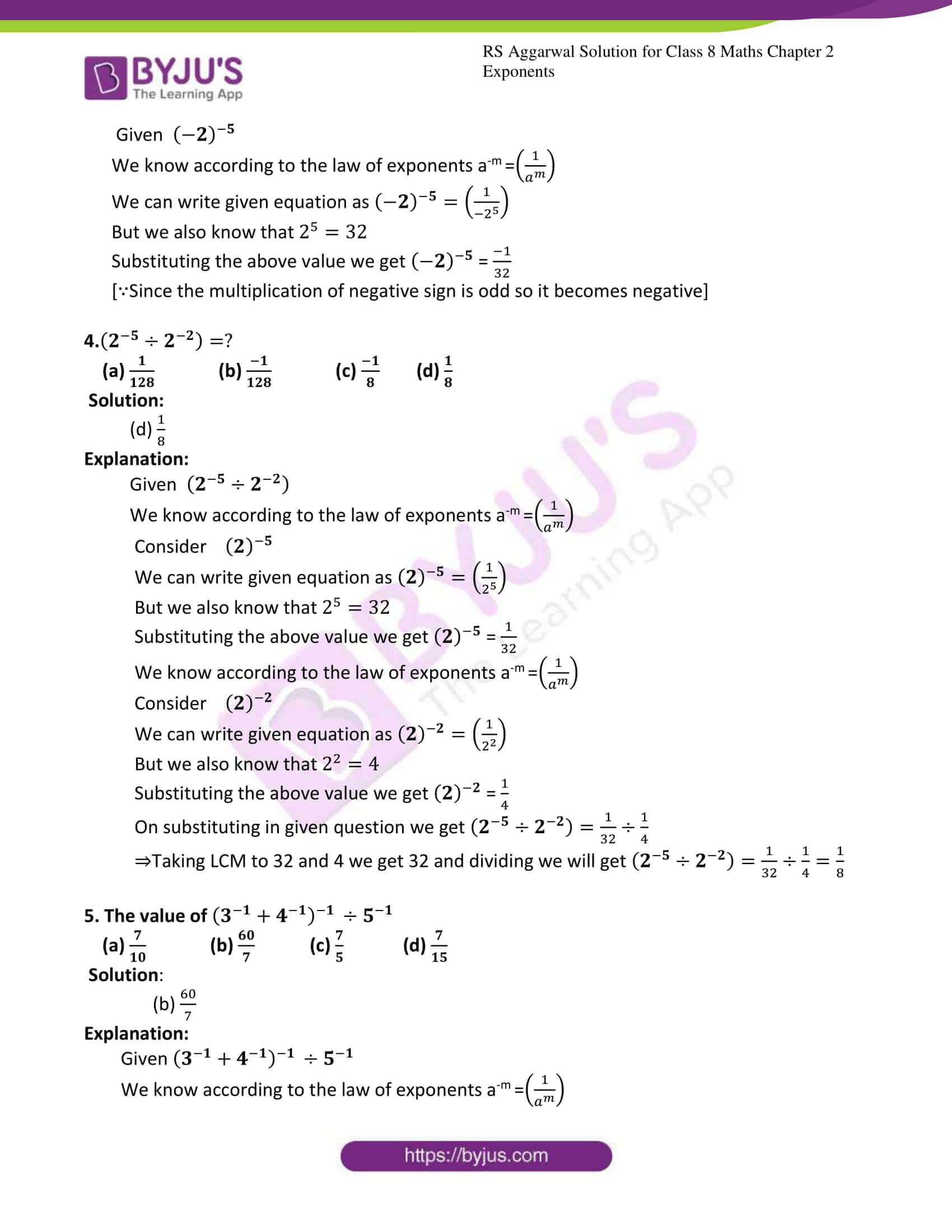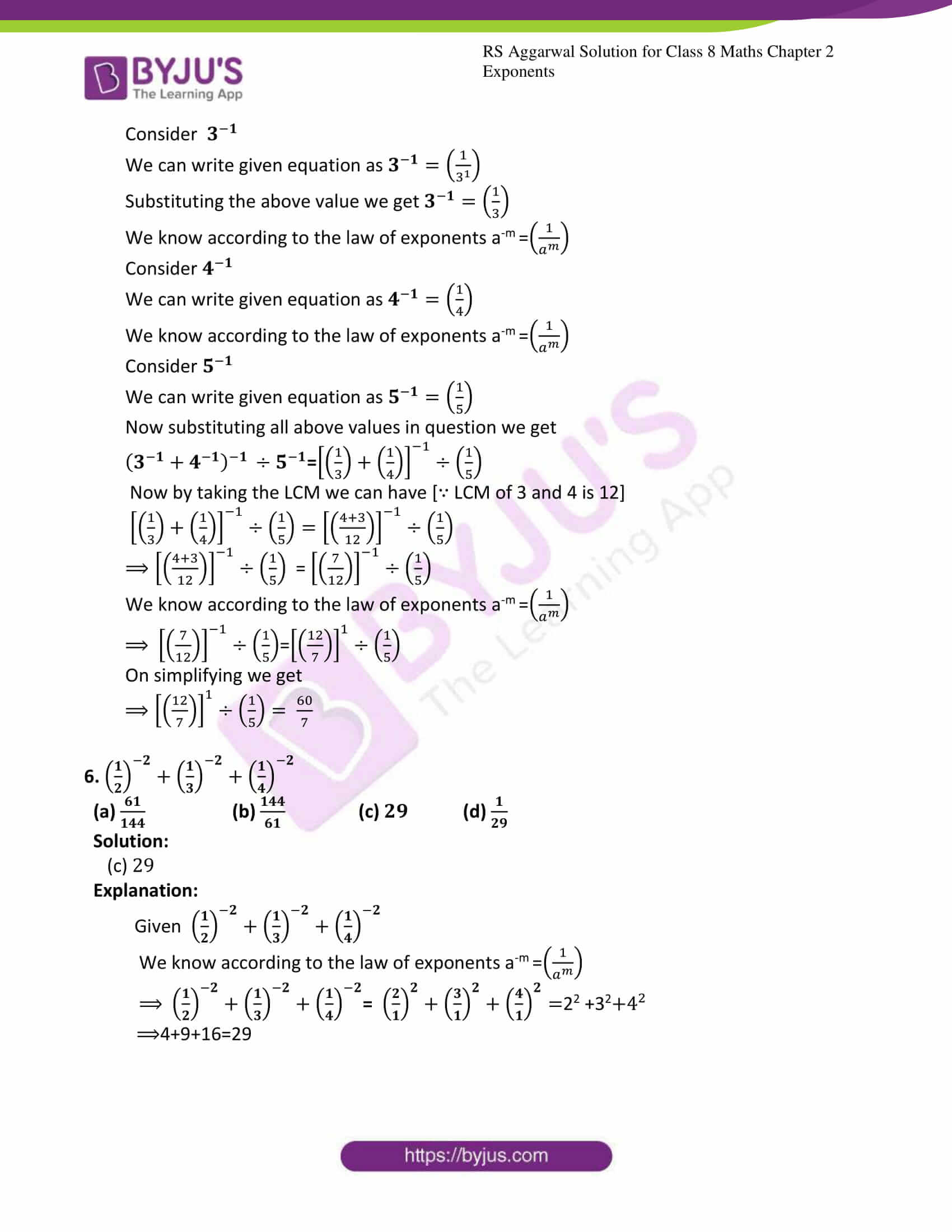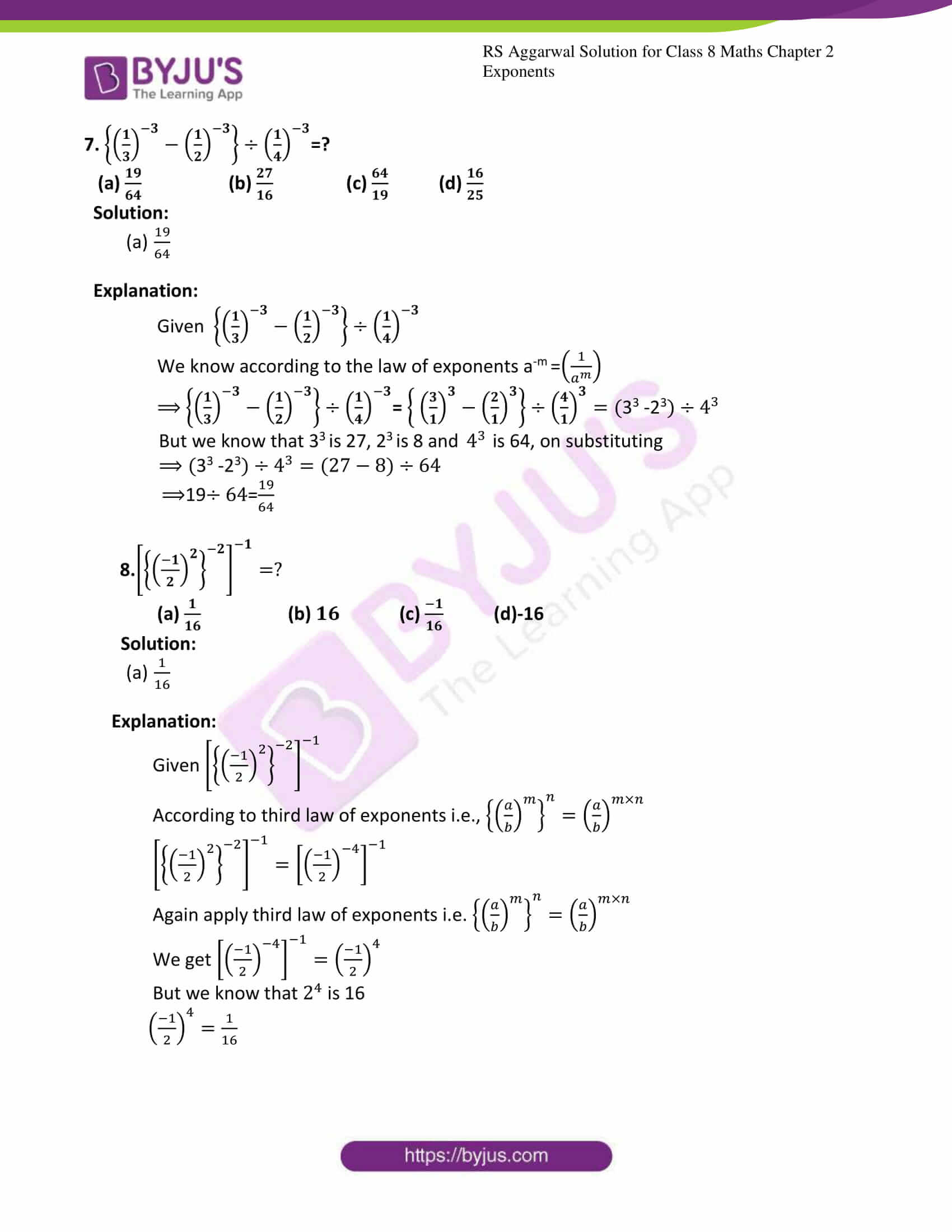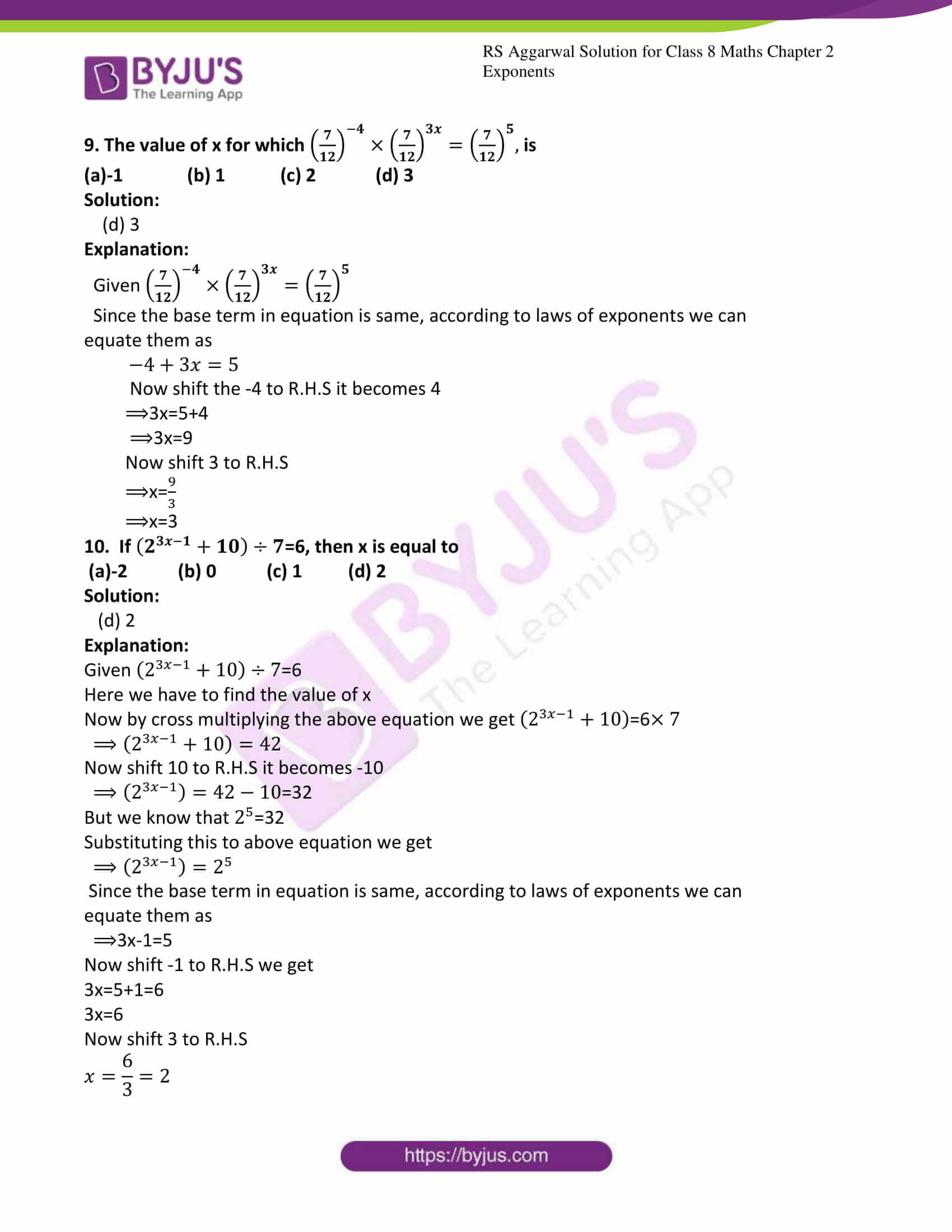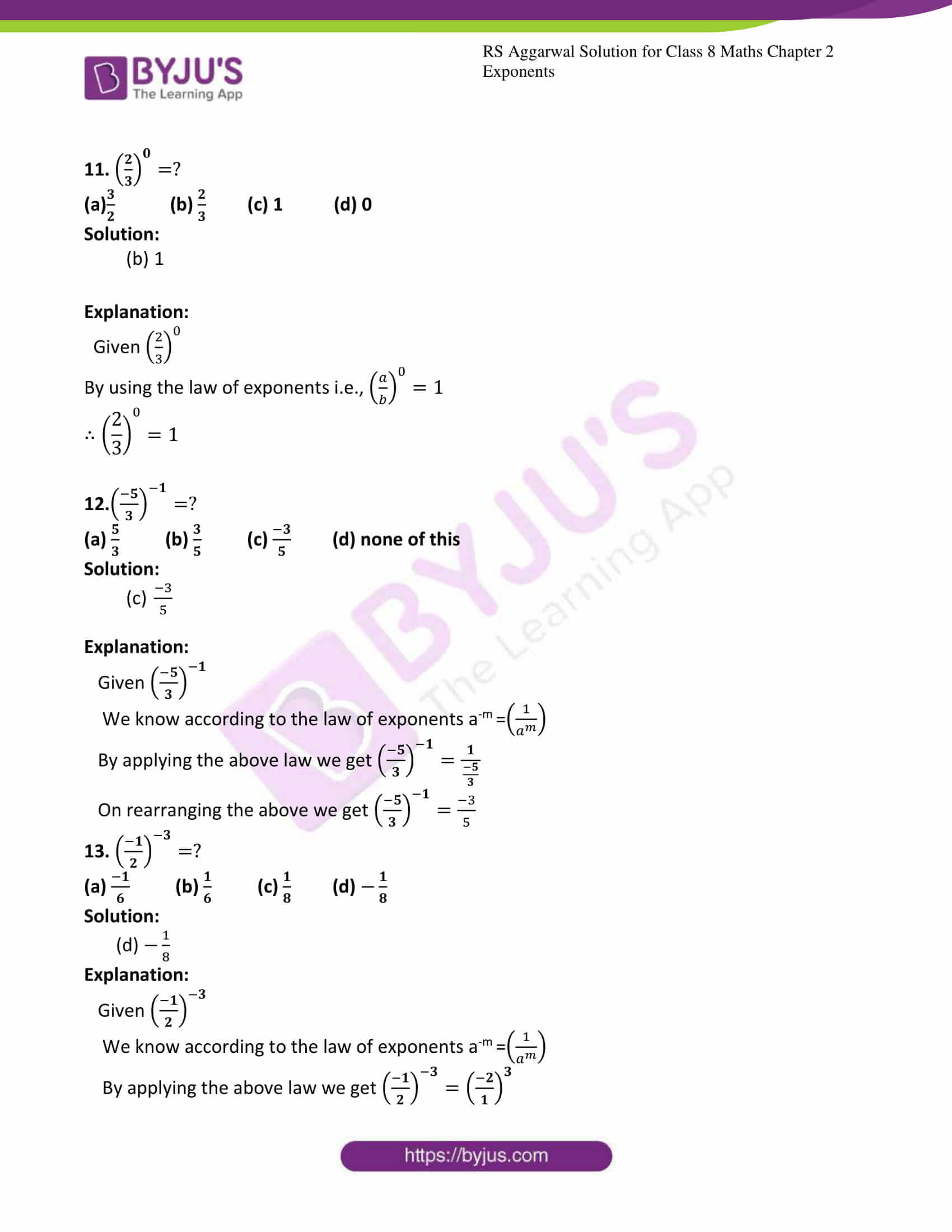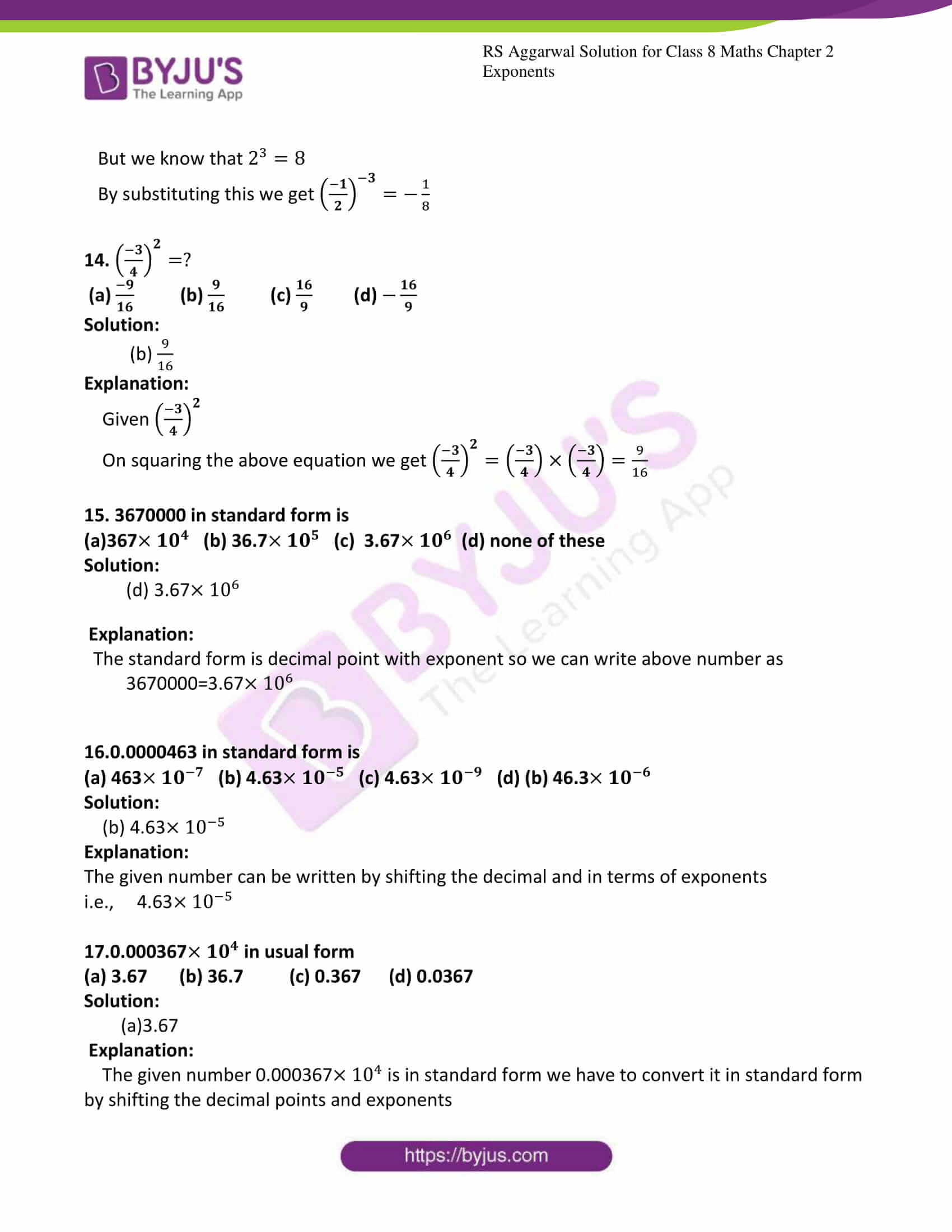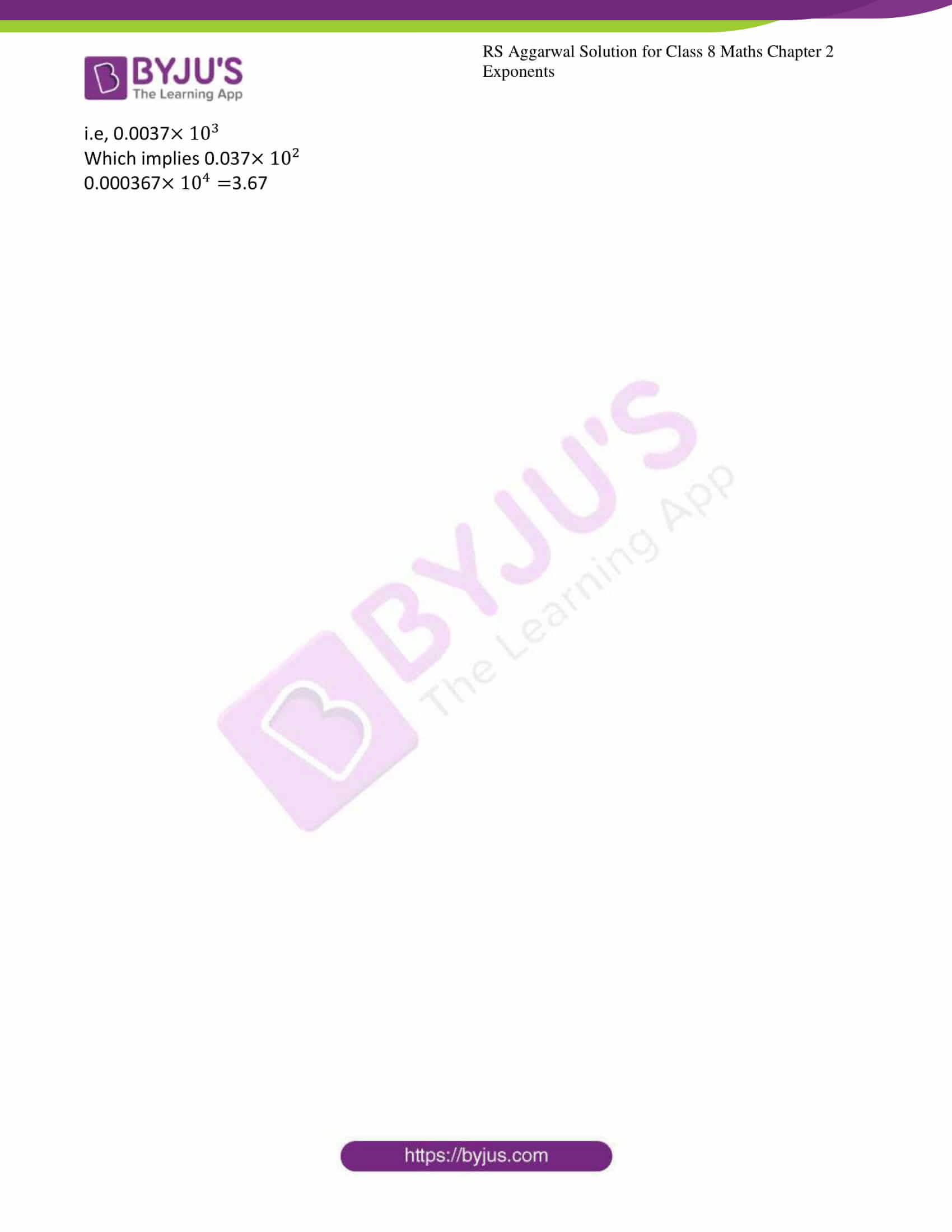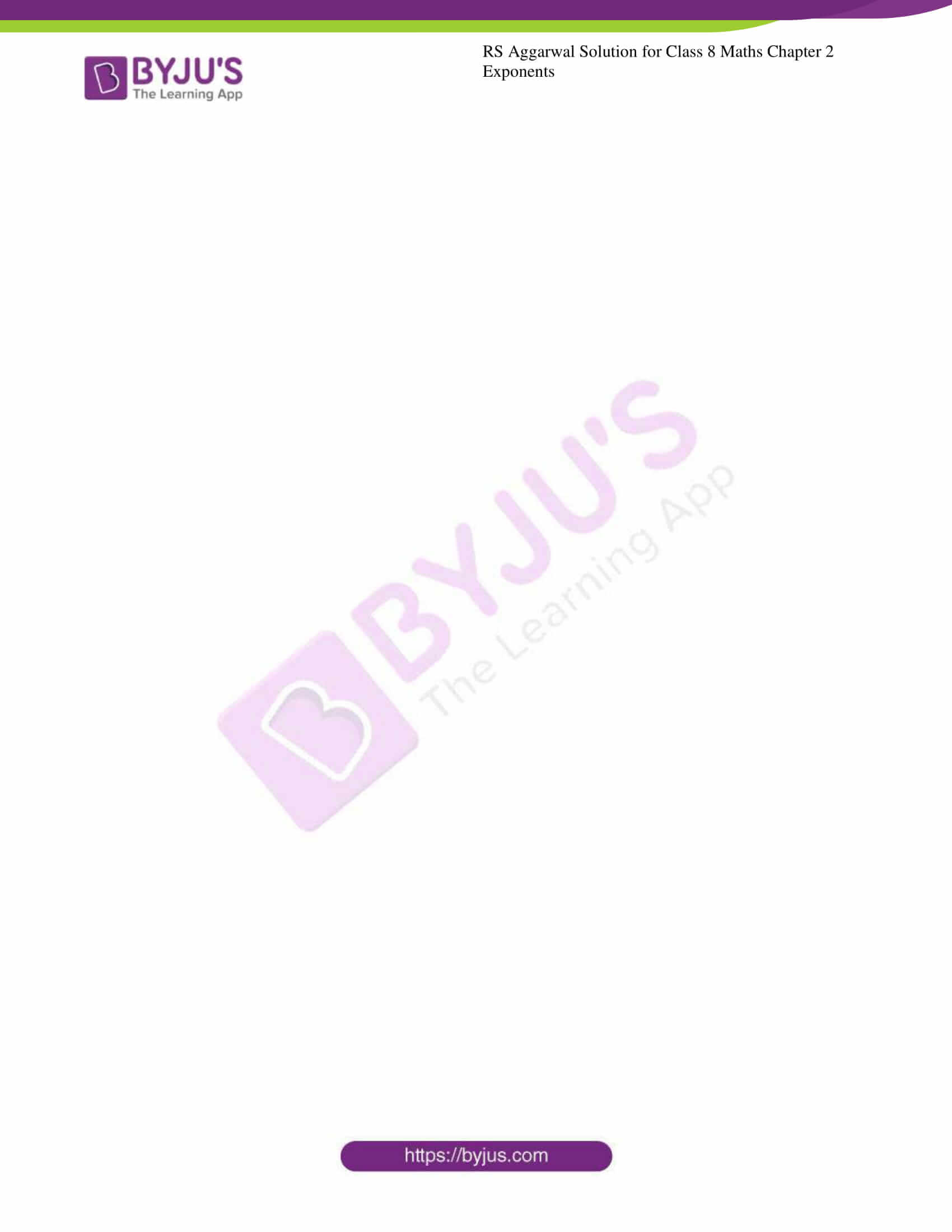### Access answers to Maths RS Aggarwal Solutions for Class 8 Chapter 2 – Exponents Exercise 2C

Select the correct answer in the each of the following: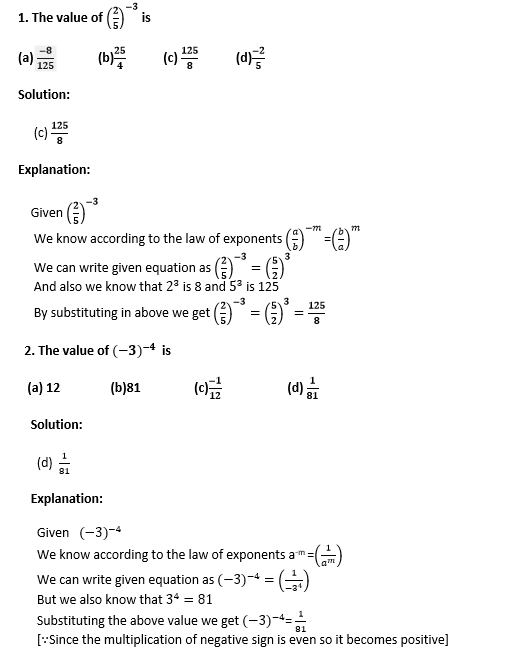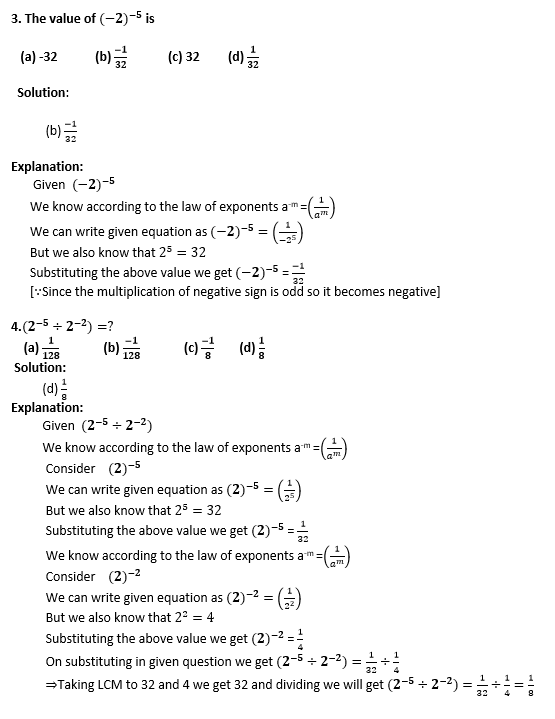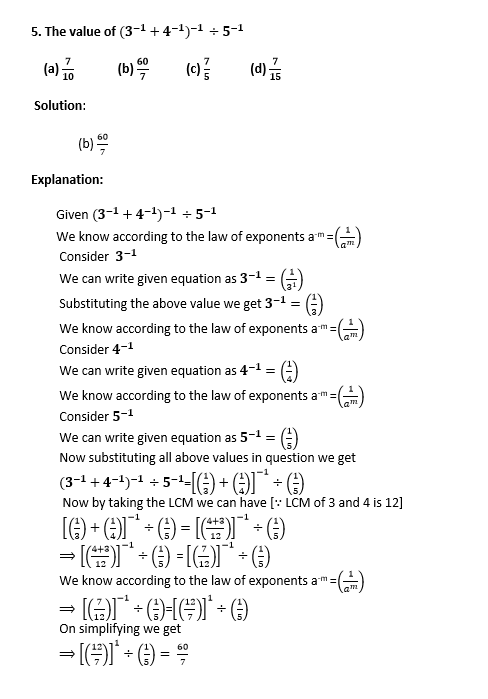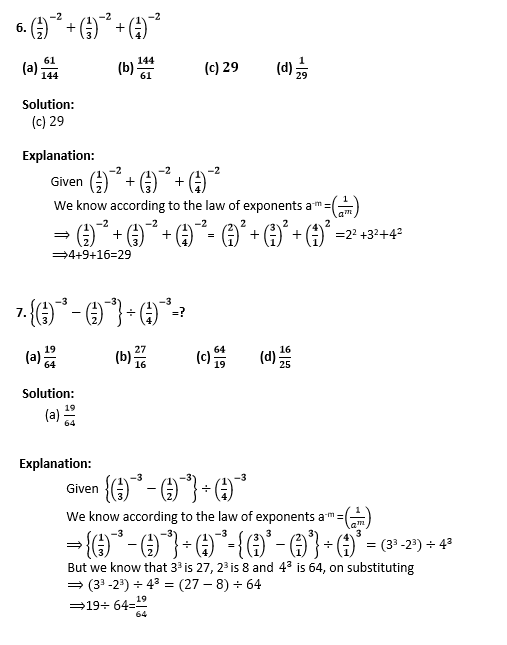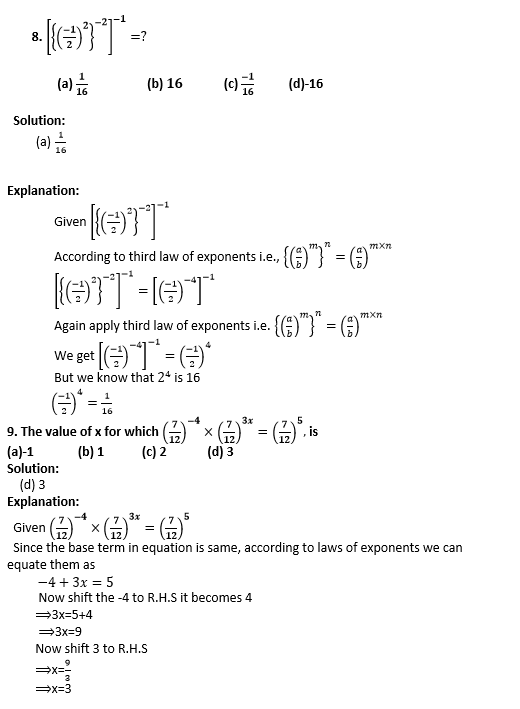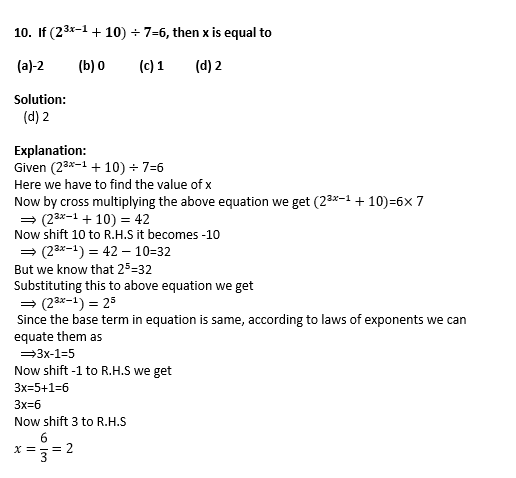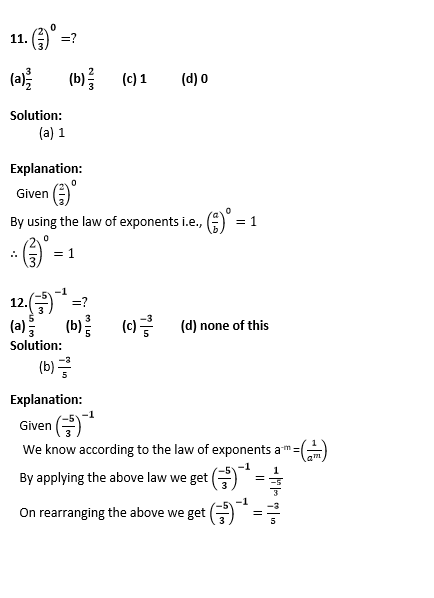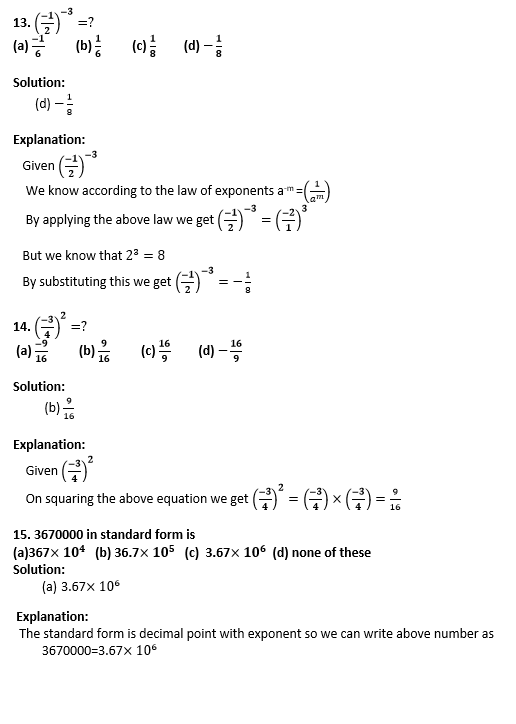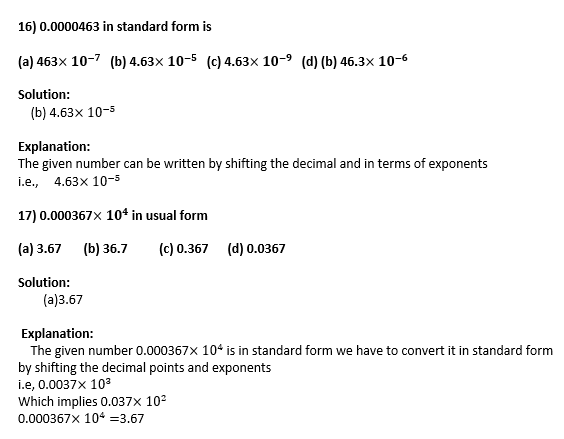## RS Aggarwal Solutions for Class 8 Maths Chapter 2 – Exponents Exercise 2C

Exercise 2C of RS Aggarwal Solutions for Chapter 2, Exponents, deals with the basic concepts related to Exponents. This exercise contains an objective type of questions. We can say that this exercise mainly deals with the laws of exponents that the students have learned in this chapter. This exercise covers all the topics of this chapter. Some of the topics focused on Exercise 2C are mentioned below.

• Positive integral of Exponent of a rational number
• The negative integral of an exponent of a rational number
• Laws of exponents
• Numbers in standard form
• Expressing very large numbers in standard form
• Expressing very small numbers in standard form

The RS Aggarwal Solutions can help the students in exercising and learning each and every concept as it provides solutions to all questions asked in the RS Aggarwal textbook. Those who aim to score high marks in Maths of Class 8 are advised to practice all the questions present in RS Aggarwal book as many times as possible.